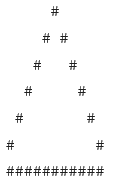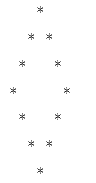Code: Hollow Diamond Pattern

# Code: Hollow Diamond Pattern - Basics of Python - Software Development

### 1. Hollow pyramid/triangle pattern

The pattern is similar to pyramid pattern. The only difference is, we will replace all internal ‘#’ or ‘*’ characters by space character and we will print 2*N-1 (N = number of rows in pattern) ‘#’ or ‘*’ characters in last row.

Examples:
Input: n=6
Output:// CPP program to print a hollow pyramid pattern

#include <iostream>

using namespace std;

void printPattern(int);

int main()

{

int n = 6;

printPattern(n);

}

void printPattern(int n)

{

int i, j, k = 0;

for (i = 1; i <= n; i++) // row=6

{

// Print spaces

for (j = i; j < n; j++) {

cout << " ";

}

// Print #

while (k != (2 * i - 1)) {

if (k == 0 || k == 2 * i - 2)

cout << "#";

else

cout << " ";

k++;

}

k = 0;

cout << endl; // print next row

}

// print last row

for (i = 0; i < 2 * n - 1; i++) {

cout << "#";

}

}

Output### 2. Hollow Diamond

Note: For even input, print the pattern for n-1.

Example:
Input: 1
Output:

*
For n=1

Input: 7
Output:Approach: To print diamond we need to print spaces before star and after the star to achieve constant increasing distance of stars.
To print the box shape we need to print ‘-‘ for i==1 (first row) & i==n (last row) and ‘|’ for j==1 (first column) and j==n (last column).

Algorithm:

• If n is odd increment n.
• Find mid=n/2.
• Traverse from 1 to mid to print upper half of the pattern (say i).
• Traverse from 1 to mid-i to print spaces for upper left most outer box (say j).
• If (i==1) print ‘*’ (since for first row we need only one star).
• else print ‘*’ and traverse from 1 to 2*i-3 to print spaces for hollow diamond (say j) and print ‘*’ after loop is over.
• Traverse from 1 to mid-i to print spaces again for upper right most outer box (say j).
• Close the loop at step 3.
• Traverse from mid+1 to n-1 to print lower half of the pattern (say i).
• Traverse from 1 to i-mid to print spaces for lower left most outer box (say j).
• If (i==n-1) print ‘*’ (since for last row we need only one star).
• else print ‘*’ and traverse from 1 to 2*(n-i)-3 to print spaces for hollow diamond (say j) and print ‘*’ after loop is over.
• Traverse from 1 to i-mid to print spaces again for lower right most outer box (say j).
• Close the loop at step 9.

#include <bits/stdc++.h>

using namespace std;

// function to print the pattern

void printPattern(int& n)

{

int i,j,mid;

if(n%2==1) //when n is odd, increase it by 1 to make it even

n++;

mid = n/2;

// upper half pattern

for(i = 1; i<= mid; i++) {

for(j = 1; j<=mid-i; j++) //print the blank spaces and outer box before star

cout<<" ";

if(i == 1) {

cout << "*";

}else{

cout << "*"; //in each line star at start and end position

for(j = 1; j<=2*i-3; j++) { //print space to make hollow

cout << " ";

}

cout << "*";

}

for(j = 1; j<=mid-i; j++) //print the blank spaces and outer box after star

cout<<" ";

cout << endl;

}

// lower half pattern

for(i = mid+1; i<n; i++) {

for(j = 1; j<=i-mid; j++) //print the blank spaces and outer box before star

cout<<" ";

if(i == n-1) {

cout << "*";

}else{

cout << "*"; //in each line star at start and end position

for(j = 1; j<=2*(n - i)-3; j++) { //print space to make hollow

cout << " ";

}

cout << "*";

}

for(j = 1; j<=i-mid; j++) //print the blank spaces and outer box after star

cout<<" ";

cout << endl;

}

}

// driver's code

int main() {

int n=7;

printPattern(n);

}

Output### 3. Hollow Diamond bounded inside a rectangular box made of horizontal and vertical dashes(-).

Write a program to Print hollow diamond pattern bound inside a box made of dash(-) and bitwise-OR(|) as shown below.

Note: For even input, print the pattern for n-1.

Example:

Input: 1
Output:

*
For n=1

Input: 7
Output:Approach: To print diamond we need to print spaces before star and after the star to achieve constant increasing distance of stars.
To print the box shape we need to print ‘-‘ for i==1 (first row) & i==n (last row) and ‘|’ for j==1 (first column) and j==n (last column).

Algorithm:

• If n is odd increment n.
• Find mid=n/2.
• Traverse from 1 to mid to print upper half of the pattern (say i).
• Traverse from 1 to mid-i to print upper left most outer box (say j).
• If (i==1) print ‘*’ (since for first row we need only one star).
• else print ‘*’ and traverse from 1 to 2*i-3 to print spaces for hollow diamond (say j) and print ‘*’ after loop is over.
• Traverse from 1 to mid-i to print upper right most outer box (say j).
• Close the loop at step 3.
• Traverse from mid+1 to n-1 to print lower half of the pattern (say i).
• Traverse from 1 to i-mid to print lower left most outer box (say j).
• If (i==n-1) print ‘*’ (since for last row we need only one star).
• else print ‘*’ and traverse from 1 to 2*(n-i)-3 to print spaces for hollow diamond (say j) and print ‘*’ after loop is over.
• Traverse from 1 to i-mid to print lower right most outer box (say j).
• Close the loop at step 9.

#include <bits/stdc++.h>

using namespace std;

// function to print the pattern

void printPattern(int& n)

{

int i,j,mid;

if(n%2==1) //when n is odd, increase it by 1 to make it even

n++;

mid = n/2;

// upper half pattern

for(i = 1; i<= mid; i++) {

for(j = 1; j<=mid-i; j++) { //print the blank spaces and outer box before star

if(i==1)

cout<<"-";

else if(j==1)

cout << "|";

else cout<<" ";

}

if(i == 1) {

cout << "*";

}else{

cout << "*"; //in each line star at start and end position

for(j = 1; j<=2*i-3; j++) { //print space to make hollow

cout << " ";

}

cout << "*";

}

for(j = 1; j<=mid-i; j++) { //print the blank spaces and outer box after star

if(i==1)

cout<<"-";

else if(j==mid-i)

cout << "|";

else cout<<" ";

}

cout << endl;

}

// lower half pattern

for(i = mid+1; i<n; i++) {

for(j = 1; j<=i-mid; j++) { //print the blank spaces and outer box before star

if(i==n-1)

cout<<"-";

else if(j==1)

cout << "|";

else cout<<" ";

}

if(i == n-1) {

cout << "*";

}else{

cout << "*"; //in each line star at start and end position

for(j = 1; j<=2*(n - i)-3; j++) { //print space to make hollow

cout << " ";

}

cout << "*";

}

for(j = 1; j<=i-mid; j++) { //print the blank spaces and outer box after star

if(i==n-1)

cout<<"-";

else if(j==i-mid)

cout << "|";

else cout<<" ";

}

cout << endl;

}

}

// driver's code

int main() {

int n=12;

printPattern(n);

}

OutputThe document Code: Hollow Diamond Pattern | Basics of Python - Software Development is a part of the Software Development Course Basics of Python.
All you need of Software Development at this link: Software Development

## Basics of Python

49 videos|38 docs|18 tests

## Basics of Python

49 videos|38 docs|18 testsExplore Courses for Software Development examSignup to see your scores go up within 7 days! Learn & Practice with 1000+ FREE Notes, Videos & Tests.
10M+ students study on EduRev
Track your progress, build streaks, highlight & save important lessons and more!
Related Searches

,

,

,

,

,

,

,

,

,

,

,

,

,

,

,

,

,

,

,

,

,

;